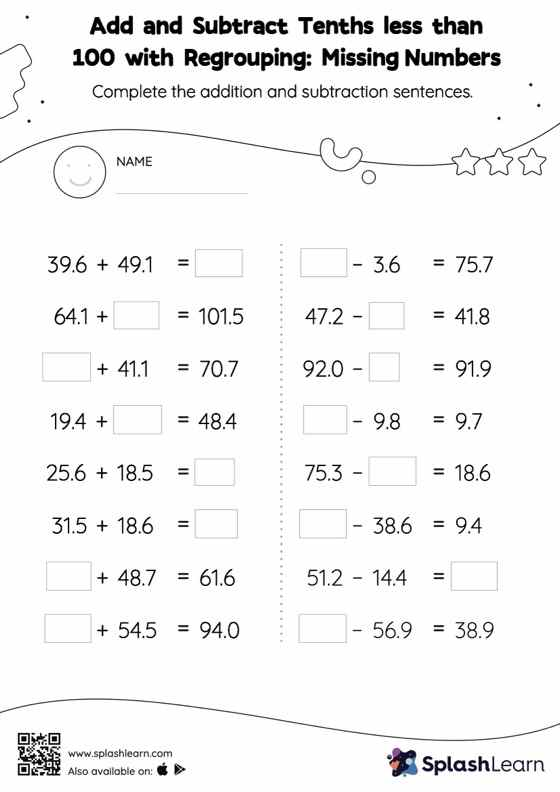# Add and Subtract Tenths less than 100 with Regrouping: Missing Numbers Worksheet

Home > Add and Subtract Tenths less than 100 with Regrouping: Missing NumbersDoes your child know how to add and subtract tenths less than 100 with regrouping? Invite them to practice this concept here. While adding and subtracting decimals, students use the relationship between addition and subtraction to find the missing number. They also need to regroup numbers in add and subtract tenths less than 100 with regrouping worksheet utilizing the relationship between ones, tenth, hundredth, thousandth, etc., To get to the result.